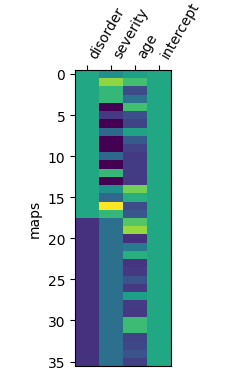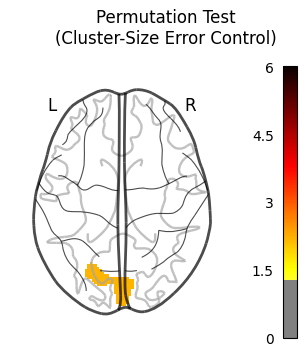# DOF in nilearn's non_parametric_inference

Hey, I am trying to create results tables for an analysis that I ran using Nilearn’s `non_parametric_inference`. And I ran into a slight inconvenience.

(If you have not tried working with Nilearn yet… stop with whatever you are doing and try it out right now, it’s awesome).

TLDR: I intend to report both uncorrected and corrected results. Since the `non_parametric_inference` function does not include uncorrected p-value map in the results output, I generated it myself with some custom code. To my surprise, I found differences in cluster sizes when I compare my uncorrected p<0.001 results with the cluster-wise corrected results from `non_parametric_inference`. I am unsure if I am running into a bug or whether I am making an error myself. So that’s why I am posting here instead of the GitHub issues page.

How I got here

My design matrix (`HCvMD_design_matrix`) looks like this:I have 2x18 brain maps, and 4 regressors. So, as far as my statistical knowledge goes, the degrees of freedom for this model is 36-4=32.

Next, I ran a non-parametric test, with a cluster threshold of 0.001:

``````HCvMD_out_dict = non_parametric_inference(
ls_imgs,
design_matrix=HCvMD_design_matrix,
model_intercept=True,
second_level_contrast="disorder",
two_sided_test=True,
smoothing_fwhm=6,
n_jobs=8,
threshold=0.001
)
``````

[insert 4m43.9s waiting music here]

After I grabbed some coffee, I first wanted to see the uncorrected results table. I used the `get_clusters_table` function. By means of trial and error (childhood habit), I found out that this function only produces sub-clusters for z-maps, and not for 10logP maps. So, I masked the t-value map from `non_parametric_inference` using cluster-size error control map, converted the map into Z values, and then produced the cluster table:

``````# Create t to z conversion function
def t_to_z(t_scores, deg_of_freedom):
p_values = scipy.stats.t.sf(t_scores, df=deg_of_freedom)
z_values = scipy.stats.norm.isf(p_values)
return z_values

# Set threshold
unc_pval=0.001
dof = len(ls_subjects) - 4 # DOF: group, covar1, covar2, intercept
threshold = stats.t.isf(unc_pval / 2, df=dof) # two-sided

# Create mask for p<0.001 uncorrected threshold
HCvMD_thr_mask_img = math_img(f'(img < {-threshold}) | (img > {threshold})', img=HCvMD_out_dict['t'])

# Convert to z-map
z_img = new_img_like(

# Create cluster table
clusters = get_clusters_table(z_img, stat_threshold=0, cluster_threshold=10, two_sided=True)
clusters = clusters.round({'X': 0, 'Y': 0, 'Z': 0})
clusters.style.format({'X': '{:,.0f}'.format,
'Y': '{:,.0f}'.format,
'Z': '{:,.0f}'.format})

``````

This gives me the following table:

Cluster ID X Y Z Peak Stat Cluster Size (mm3)
0 1 4 -86 10 4.876244 5552
1 1a -18 -78 8 4.592168
2 2 20 -76 8 4.810282 1312
3 3 16 -20 -4 3.854187 496
4 4 -40 -74 -50 3.754792 152
5 5 -8 -16 -0 3.725331 96
6 1 52 -66 14 -3.508029 96

Now, in theory, if I find any significant clusters using the cluster-size error control p-map, the cluster sizes of these surviving clusters should be the same, since I used a threshold of 0.001 (two-sided) in both cases. Right?

The resulting cluster-wise error corrected contrast looks like this:Oh cool! I found something!.This could be the first cluster from the table above. (Obviously I know MNI coorinates from the top of my head, like everyone else).

Let’s have a look…

``````# Create mask for p<0.05 cluster-size error corrected

# Convert to z-map
z_img = new_img_like(

# Create cluster table
clusters = get_clusters_table(z_img, stat_threshold=0, cluster_threshold=10, two_sided=True)
clusters = clusters.round({'X': 0, 'Y': 0, 'Z': 0})
clusters.style.format({'X': '{:,.0f}'.format,
'Y': '{:,.0f}'.format,
'Z': '{:,.0f}'.format})
``````

(yes, I know, I could’ve used more infomative variable names, but this was my quick and dirty attempt…)

This gives me the following table:

Cluster ID X Y Z Peak Stat Cluster Size (mm3)
0 1 4 -86 10 4.876244 5744
1 1a -18 -78 8 4.592168

Ah, that’s the same cluster indeed! However… everything looks the same except for the cluster size.

I figured that this must have something to do with the initial thresholding. So, I started changing the DOF value in my uncorrected result (that childhood habit again…) and found the exact same cluster size result when I changed `dof = len(ls_subjects) - 4` to `dof = len(ls_subjects) - 2`.

So, now I am wondering what’s going wrong. Did I make a mistake in my assumptions? Or is there something else going on?

In the `permuted_ols` source code, I see that covariates are first regressed out of the data before the permutation tests are being run on the residuals (correct me if I’m wrong). And if I run through the code with a debugger, the code uses 34 DOF instead of 32 DOF. In the line `dof = n_samples - (n_regressors + n_covars)`, `n_regressors` is set to 1 and `n_covars` is also set to 1 but I do not understand why.

Do you have any idea @ymzayek?

Interesting. Let me have a look.

1 Like

My understanding is that when `non_parametric_inference` calls `permuted_ols`, it just provide the column of the design matrix of interest (nilearn/second_level.py at df62edf5136b94749d8ab99abdbf210db5e7c799 · nilearn/nilearn · GitHub), and forgets to provide the other columns as covariates.
then `permuted_old` only sees a one-columns deign matrix + an intercept. It misses the other columns.
Does that makes sense ?
This is a bug. Can you open an issue on Nilearn ?
Thx,
Bertrand

1 Like

Ah thanks for looking and pointing out where the problem lies. I might have some time in the coming days to see if I can make a PR too then.

1 Like